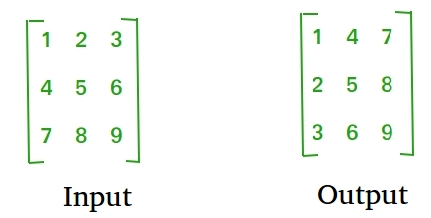Open in App
Not now

# Python Program to find transpose of a matrix

• Difficulty Level : Easy
• Last Updated : 23 Jun, 2022

Transpose of a matrix is obtained by changing rows to columns and columns to rows. In other words, transpose of A[][] is obtained by changing A[i][j] to A[j][i].For Square Matrix: The below program finds transpose of A[][] and stores the result in B[][], we can change N for different dimension.

## Python3

 `# Python3 Program to find``# transpose of a matrix` `N ``=` `4` `# This function stores``# transpose of A[][] in B[][]` `def` `transpose(A,B):` ` ``for` `i ``in` `range``(N):``  ``for` `j ``in` `range``(N):``   ``B[i][j] ``=` `A[j][i]` `# driver code``A ``=` `[ [``1``, ``1``, ``1``, ``1``],`` ``[``2``, ``2``, ``2``, ``2``],`` ``[``3``, ``3``, ``3``, ``3``],`` ``[``4``, ``4``, ``4``, ``4``]]`  `B ``=` `A[:][:] ``# To store result` `transpose(A, B)` `print``(``"Result matrix is"``)``for` `i ``in` `range``(N):`` ``for` `j ``in` `range``(N):``  ``print``(B[i][j], ``" "``, end``=``'')`` ``print``()`` ` `# This code is contributed by Anant Agarwal.`

Output:

```Result matrix is
1  2  3  4
2  2  3  4
3  3  3  4
4  4  4  4```

Time Complexity: O(n2)
Auxiliary Space: O(n2)

For Rectangular Matrix: The below program finds transpose of A[][] and stores the result in B[][].

## Python3

 `# Python3 Program to find``# transpose of a matrix` `M ``=` `3``N ``=` `4` `# This function stores``# transpose of A[][] in B[][]` `def` `transpose(A, B):` ` ``for` `i ``in` `range``(N):``  ``for` `j ``in` `range``(M):``   ``B[i][j] ``=` `A[j][i]` `# driver code``A ``=` `[ [``1``, ``1``, ``1``, ``1``],`` ``[``2``, ``2``, ``2``, ``2``],`` ``[``3``, ``3``, ``3``, ``3``]]`  `# To store result``B ``=` `[[``0` `for` `x ``in` `range``(M)] ``for` `y ``in` `range``(N)]` `transpose(A, B)` `print``(``"Result matrix is"``)``for` `i ``in` `range``(N):`` ``for` `j ``in` `range``(M):``  ``print``(B[i][j], ``" "``, end``=``'')`` ``print``() `

Output:

```Result matrix is
1  2  3
1  2  3
1  2  3
1  2  3```

Time Complexity: O(n*m)
Auxiliary Space: O(n*m)

In-Place for Square Matrix:

## Python3

 `# Python3 Program to find``# transpose of a matrix` `N ``=` `4` `# Finds transpose of A[][] in-place``def` `transpose(A):` ` ``for` `i ``in` `range``(N):``  ``for` `j ``in` `range``(i``+``1``, N):``   ``A[i][j], A[j][i] ``=` `A[j][i], A[i][j]` `# driver code``A ``=` `[ [``1``, ``1``, ``1``, ``1``],`` ``[``2``, ``2``, ``2``, ``2``],`` ``[``3``, ``3``, ``3``, ``3``],`` ``[``4``, ``4``, ``4``, ``4``]]` `transpose(A)` `print``(``"Modified matrix is"``)``for` `i ``in` `range``(N):`` ``for` `j ``in` `range``(N):``  ``print``(A[i][j], ``" "``, end``=``'')`` ``print``()`` ` `# This code is contributed by Anant Agarwal.`

Output:

```Modified matrix is
1  2  3  4
1  2  3  4
1  2  3  4
1  2  3  4```

Time Complexity: O(n2)
Auxiliary Space: O(1)

Please refer complete article on Program to find transpose of a matrix for more details!

My Personal Notes arrow_drop_up